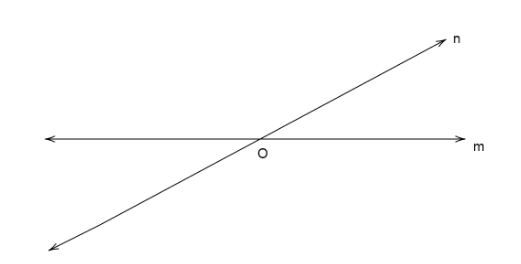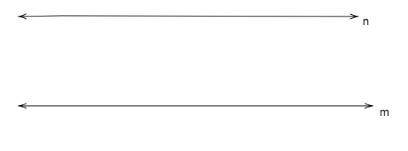QuestionAnswers

# State true or false.Lines which meet at a common point are called parallel lines.A. TRUEB. FALSEVerified
128.4k+ views
Hint: Here we use the definition of parallel lines and a diagram to show a point of intersection of two lines and check that the two intersecting lines are parallel to each other or not.

We take two lines m and n which meet at a point O.
Two lines are said to have a common point when they meet each other at the point which is called point of intersection. Here the common point is O.We know from the definition of parallel lines that two lines are said to be parallel if they never intersect or they never meet even if extended in any direction i.e. the two lines m and n are always the same distance apart.
Since the figure of two intersecting lines show that they intersect at a point O, therefore the lines m and n cannot be parallel as the set of lines intersect at point O.
Therefore, the statement in the question is FALSE.
So, option B is correct.

Note:
* Parallel lines are written with a sign $\parallel$between them which is written as $m\parallel n$.
* If two lines are parallel to a single line, then the three lines are said to be parallel to each other.
* Parallel lines m and n can be drawn asStudents might give wrong answers if they think lines starting from point O never meet so they are parallel which is wrong because the set of lines still meets at point O which is the point of intersection.